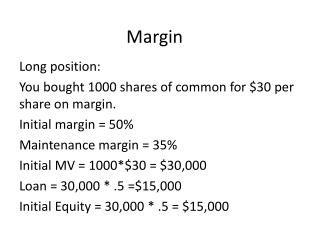DownloadDownload PresentationMargin

# Margin

Télécharger la présentation## Margin

- - - - - - - - - - - - - - - - - - - - - - - - - - - E N D - - - - - - - - - - - - - - - - - - - - - - - - - - -
##### Presentation Transcript

1. Margin Long position: You bought 1000 shares of common for \$30 per share on margin. Initial margin = 50% Maintenance margin = 35% Initial MV = 1000*\$30 = \$30,000 Loan = 30,000 * .5 =\$15,000 Initial Equity = 30,000 * .5 = \$15,000

2. If price went up to \$40 what is: Your equity? New Equity = new MV – Loan = \$40 *1000 -15,000= \$25,0000 Your profit? Profit = New Equity – Initial equity = 25,000 -15,000 = \$10,000 % of margin? Margin% = new equity/ MV = 25000/40,000 = .625

3. At what price below which you receive margin call?

4. P= 15000/1000 (1- .35) = \$23.08 If price falls to \$20: What is your equity? New Equity = new MV – Loan = 20000- 15,000 = 5000 What is your profit ? Profit = New equity – initial equity = 5000 – 15000 = -\$10,000 What is your margin? Margin% = equity/ MV = 5000/20,000 = .25

5. When the price is \$20, how much you need to deposit with your broker to restore maintenance margin requirement? Margin % = Equity needed/newMV .35 = Equity/ 20,000 Equity needed = \$7,000 Cash requested = equity needed – new equity = 7,000 – 5,000 = \$2,000

6. Margin, Short sell You sell 2000 shares of common stock at \$40 per share short. Initial margin is 40%. Maintenance margin is 35%. Initial Equity = 80,000 * .4 = 32000 Loan = 80,000 * .6 = 48,000 Initial cash with your broker = proceeds from the sale of stock + initial equity = 80,000+ 32,000 = 112,000

7. How far the price of stock can go up before getting a margin call? P= 112,000/2000(1+.35) = \$41.48

8. If price goes up to \$45, What is your new equity? New Equity = Initial cash – new MV New Equity = 112,000 – 90,000 =22,000 What is profit? Profit = New equity – initial equity =22,000- 32000 = -\$10,000 What is your new margin? New margin = New equity/ new MV = 22000/90000 = .2444

9. When the price is \$45, how much cash needs to be deposited with your broker to meet maintenance margin requirements? Maintenance margin = equity needed / new MV .35 = equity needed/90000 Equity needed = 31500 Cash requested = equity needed – new equity 31,500 – 22,000 = \$9,500

10. If price falls to \$30, What is your new equity? New equity = Initial cash – New MV = 112,000 – 60,000 = 52,000 What is your profit? Profit = New equity – initial equity = 52000 – 32000 = \$20,000